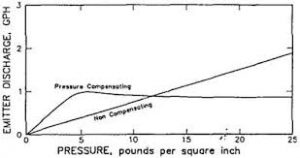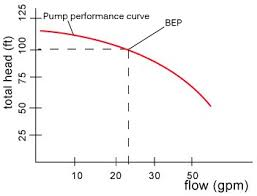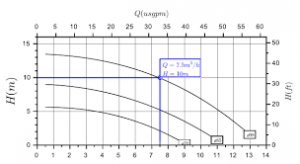## How to Calculate and Solve for Emitter Discharge | Irrigation Water RequirementThe image above represents emitter discharge.

To compute for emitter discharge, three essential parameters are needed and these parameters are Constant for Each Emitter (K), Pressure Head (h) and Emitter Discharge Exponent (x).

The formula for calculating emitter discharge:

q = Khx

Where:

q = Emitter Discharge
K = Constant for Each Emitter
x = Emitter Discharge Exponent

Let’s solve an example;
Find the emitter discharge when the constant for each emitter is 21, the pressure head is 12 and the emitter discharge exponent is 2.

This implies that;

K = Constant for Each Emitter = 21
h = Pressure Head = 12
x = Emitter Discharge Exponent = 2

q = Khx
q = (21)(12)2
q = (21)(144)
q = 3024

Therefore, the emitter discharge is 3024.

## How to Calculate and Solve for Total Head | Aquifer CharacteristicsThe image above represents total head.

To compute for total head, two essential parameters are needed and these parameters are Pressure head (hpand Elevation head (z).

The formula for calculating total head:

h = hp + z

Where:

Let’s solve an example;

This implies that;

hp = Pressure Head = 21
z = Elevation Head = 32

h = hp + z
h = 21 + 32
h = 53

Therefore, the total head is 53.

hp = h – z

Where;

Let’s solve an example;
Given that the total head is 34 and the elevation head is 20. Find the Pressure head?

This implies that;

h = Total Head = 34
z = Elevation Head = 20

hp = h – z
hp = 34 – 20
hp = 14

Therefore, the pressure head is 14.

## How to Calculate and Solve for Pressure Head | Aquifer CharacteristicsThe image above represents pressure head.

To compute for pressure head, three essential parameters are needed and these parameters are Pressure (P), Density (ρ) and Acceleration due to Gravity (g).

The formula for calculating pressure head:

hp = P / ρg

Where:

P = Pressure
p = Density
g = Acceleration due to Gravity

Let’s solve an example;
Find the pressure head when the pressure is 18, the density is 14 and the acceleration due to gravity is 9.

This implies that;

P = Pressure = 18
p = Density = 14
g = Acceleration due to Gravity = 9

hp = P / ρg
hp = 18 / (14)(9)
hp = 18 / 126
hp = 0.142

Therefore, the pressure head is 0.142.

Calculating the Pressure when the Pressure Head, the Density and the Acceleration due to Gravity is Given.

P = hp x pg

Where;

P = Pressure
p = Density
g = Acceleration due to Gravity

Let’s solve an example;
Find the pressure when the pressure head is 25, the density is 6 and the acceleration due to gravity is 9.

This implies that;

hp = Pressure Head = 25
p = Density = 6
g = Acceleration due to Gravity = 9

P = hp x pg
P = 25 x (6)(9)
P = 25 x 54
P = 1350

Therefore, the pressure is 1350.